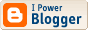<\$BlogRSDURL\$>

The Nacreous Oughts

## Kapteyn's Star

Two planets have just been discovered around nearby Kapteyn's Star, an ancient metal-poor subdwarf in the constellation Pictor. With a visual magnitude of 8.853 and a parallax of "0.25566, the luminosity works out to 0.003832431762. Trying to match the radius as given, i figure this MO star with BC -1.0925 and T= 3450 K, for a total brightness of 0.01048273 solar, which gives a radius of 0.289370994.

Using a stellar mass of 0.274, the inner planet whose period is 48.616 days orbits at 0.169318524 AU, and the outer planet, at 121.54 days, would be 0.311887389. (Roughly this represents an orbital resonance of 12 to 5.) The corresponding blackbody temperatures are 217 and 160 K. However, their orbits are rather eccentric (0.21 & 0.23), so these numbers vary from 198 to 245 and 145 to 183. So even the inner planet is rather colder than Earth.

The tidal effect on these planets is 9.5 and 2.8, which leads to the consideration that the inner planet would either be captured, like several other "habitable-zone" exoplanets we have seen, or very long; by a rather complicated rule of thumb I have devised, i find the solar day of these worlds 674 days (22 months) long, and 10 days long, respectively. That would cause extreme temperature variations in the warmer world, moderated of course by the amount of atmosphere it possesses. But we know very little of what to expect with planets of a metal-poor, halo star such as this. One can extrapolate that the absence of heavy elements would result in a less dense planet overall; for instance, with metallicity (log Fe/H -0.86) of 0.138038426, it might be something like a fourth of Earth's. So the inner planet, with 4.8 times Earth's mass, might have a radius of 2.8 and a gravity of 0.63; the outer one, with 7.0 Earth's mass, R= 3.2 and G= 0.71.

If i assume the albedo of each world is 0.4375 and 0.51, and the greenhouse effect 50 and 60, the average temperatures (excluding diurnal variation), amount to -31.5, plus or minus 20.5 (due to orbital eccentricity), and -76 plus or minus 16 C. The outer planet might vary 10 degrees more either way if its solar day is 10, while the inner planet could vary by a hundred degrees more in the daytime, and less at night, with the slowly-rotating model. This is not a mild climate. It might be possible to find an atmospheric pressure such that water does not boil; certainly it would freeze long before sunset... The outer planet seems to be a bit too cold for an ammonia world; perhaps sulfur dioxide might work as a solvent there.

In addition to these changes, the star itself is a BY Draconis variable with a range of 8.90 to 9.22 magnitude; the variation is quasiperiodic, linked to the star's rotation period (195 days).

I am naming planet b Absamon, and planet c Maccasor (both names from the Sworn Book of Honorius). At closest approach (some 0.035 AU) each would show a visible disk, perhaps 1/4th the size of a full Moon as seen from Earth. From Absamon, Maccasor would have a synodic period of 81.0 days--a little less than an eighth of its solar day--but i would expect some kind of synchronization to have occurred.

[A synchronization of exactly 8 to 1 would make the rotation period 46.38 days, for a solar day of 1010 days. (7/14) --It takes 4 days for its sun to clear the horizon.]

------------------------------------------ Follow-up. And.

Labels: